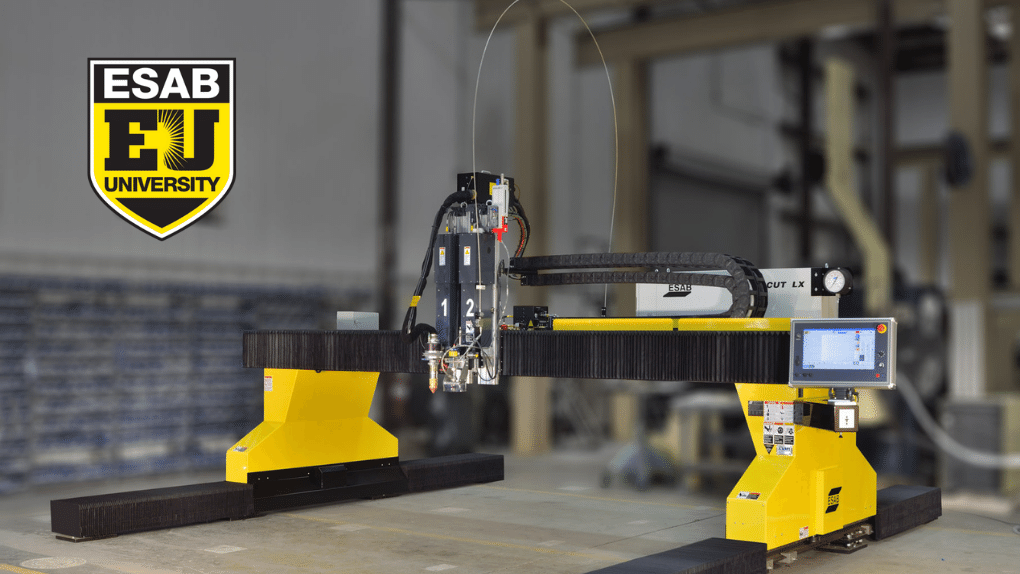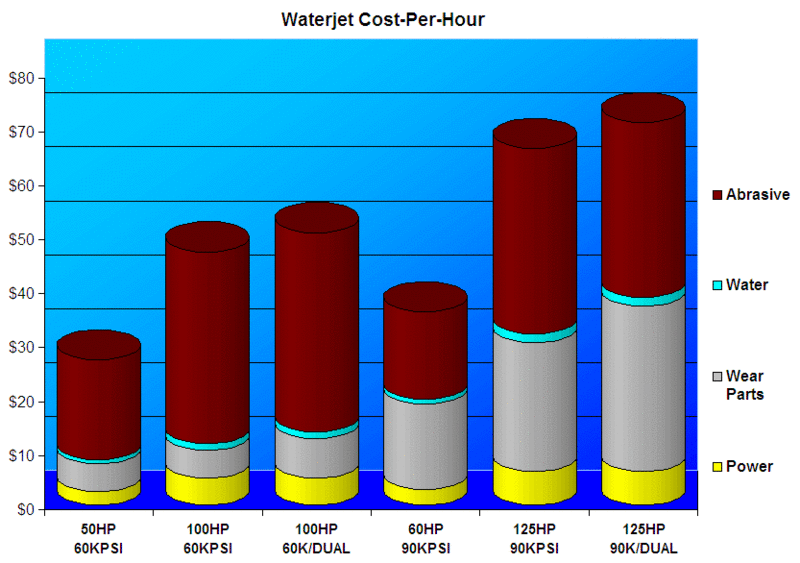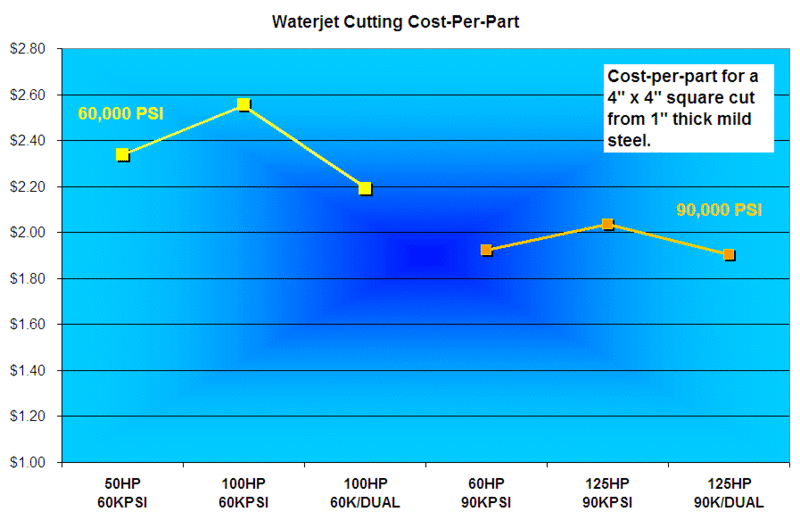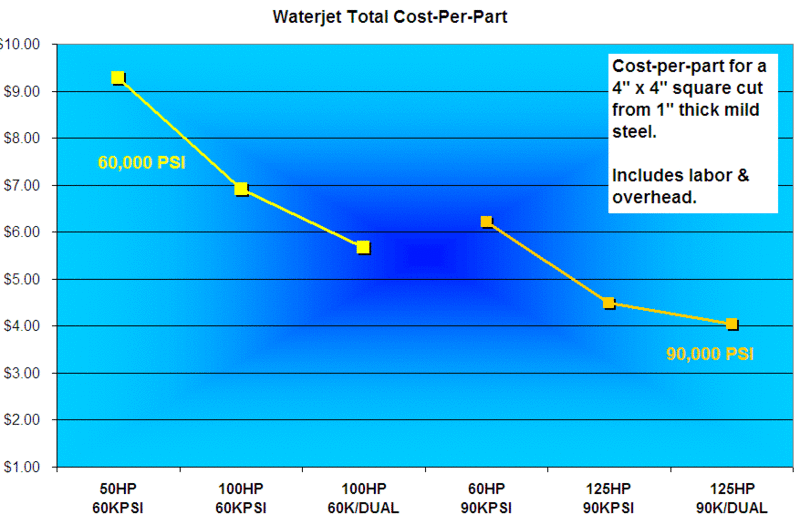# How Much Does Waterjet Cutting Cost?

## What Is The Operational Cost Of Waterjet Cutting?

The cost to operate a waterjet is a common question, as people struggle to understand the difference between running a typical plasma cutting machine and a waterjet cutter. There are different consumables, different power requirements, cost of water, and most importantly, the cost of abrasive. Garnet abrasive can account for as much as 75 percent of the total hourly operating cost of a waterjet cutting system. So, understanding what drives its use and how much it costs will be important to the customer considering a waterjet machine.

To dig into this question a little deeper, let us look at six different waterjet system configurations, including:

1. 60,000 PSI (Per Square Inch) / 50 HP (High Pressure) pump
2. 60,000 PSI / 100 HP pump
3. 60,000 PSI / 100 HP pump running two cutting heads
4. 90,000 PSI / 60 HP pump
5. 90,000 PSI / 125 HP pump
6. 90,000 PSI / 125 HP pump running two cutting heads.

For each of these, the cost of power, wear parts, water, and abrasive, on a cots per hour basis, as shown in the chart below. Each of these costs represents the maximum water and abrasive flow rate for the largest orifice that can be driven by each pump at its maximum pressure.If you take the same data and look at each number as a percentage of overall cost, it looks like this:

### How Much Does Waterjet Cutting Cost?

We need to take all the operational components into account to understand what makes up the cost of waterjet cutting. The various operational components involved in waterjet cutting include:

#### Cost of water

The smallest slice of the pie is the cost of water. I am using a national average of \$3.51 per 1000 gallons. Let us include both maximum cutting water flow and cooling water for the hydraulics. The cost would range between \$1,200 and \$2,500 per year. That does not consider any additional cost for sewer, so depending on your locale, that cost could be higher.

#### Cost of power

The next chunk of the cost is the power to run the intensifier pump. Assuming an average of 7.46 cents per KWh, the cost would range between \$3,700 and \$9,400 per year. This assumes the pump is running 6 hours per day and looking at pumps between 50HP and 125HP.

#### Cost of wear parts

The next biggest cost is the wear parts. This includes replacing the orifice, focusing tubes, pump seals, and the cost of miscellaneous pump parts calculated out on a cost per hour basis. This is going to range between \$5 and \$22 per hour, so using the same assumptions, it will total up to anywhere from \$7,700 to \$32,000 per year.

#### Cost of abrasive

The biggest chunk of the cost comes from the abrasive. Typical garnet abrasive will cost around 22 to 30 cents per pound, depending on the type of abrasive, purchasing volume, and negotiated discount.

There are two main types of garnet: hard rock and alluvial. Hard rock or crushed rock is crushed down rock to a specific mesh size from larger chunks of garnet rock. Hard rock garnet is sharper, and therefore typically cuts a little faster. Alluvial garnet is mined from beaches and riverbeds, already worn down to sand by natural forces. Therefore, its grains are rounded and cut a little bit slower. This makes hard rock abrasive expensive than alluvial. For an average, let us consider 25 cents per pound.

We need to consider the abrasive flow rate as well. Most metal plate cutting is going to use somewhere between 1 to 2.3 pounds per minute. Therefore, on average, you are going to be spending between \$18 to \$36 per hour on abrasive. Assuming 6 hours of cutting time per day, that is \$110 to \$220 of abrasive per day, or \$550 - \$1,100 per week, \$2,200 to \$4,400 per month, \$26,000 to \$55,000 per year.

Now, to make these numbers useful, we need to consider the differences in cutting speed, so we can calculate a cost-per-inch, or cost-per-part, which is much more useful in comparing one process to the next. For this calculation, let us assume a 4” x 4” part cut from 1” thick mild steel, for a total of 16” of cut length. The resulting cost-per-part is shown below.The actual values will change depending on the material type and part size, but the relative cost will remain the same. The above chart looks as if it is useful to illustrate the cost savings advantage of higher-pressure pumps: even though the actual cost-per-hour is higher, the resulting cost-per-part is lower.

However, one important piece of the puzzle is still missing, that will make a significant difference. The above chart does not include labor, overhead, or burden rates. Once an hourly rate is included for those factors, we can calculate the difference in ROI (return on investment) from one type of pump to the next, which helps justify the purchase of the higher-priced pumps.

The below chart displays an hourly rate to represent labor and overhead. The rate is spread over the total number of parts that could be cut in a period. The resulting chart below shows how the increased speed of cut with the higher pressure pumps impacts the overall cost.Of course, the calculations do not have to stop here. Various other crucial factors also need to be taken into consideration. The increased number of parts that can be cut in a day, and the increased profit that could be made from those parts. We also need to factor in the difference in equipment cost, all leading toward a final ROI calculation. But that will have to be the subject of a future post.